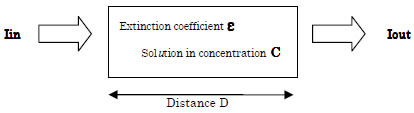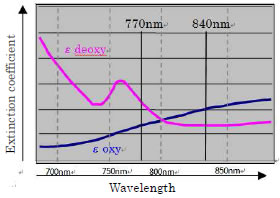1) Acquisition principle of biological information

Based on the Beer-Lambert law, assuming that the incident light intensity to a solution in a certain density is Iin, and the light intensity that got through the solution is Iout, it is known that the following expression is true.-Log(Iout / Iin) = ε x C x D

That is, if molecular absorption coefficient ε of the solution in a specific wavelength was obtained beforehand, the concentration C of the solution can be obtained by measuring Iin, Iout, and D.

The following is the modified Beer-Lambert Law, that is applied to a medium with scattering by extending the Beer-Lambert Law

-Log(Iout / Iin) = ε x C x D + S

Here, S represents the attenuation of the light intensity by scattering. D represents the average optical path length.

When in solution concentration (solute) is changed to C + ΔC from C, the amount of transmitted light has changed to Iout + ΔIout, it becomes

-Log[ (Iout + ΔIout) / Iin] = ε x (C + ΔC) x D + S

When the attenuation S of scattering light intensity does not change, it is shown by the formula from two equations above

Log[ (Iout + ΔIout) / Iout] = ε x ΔC x D

Of the incident light intensity to live body in a specific wavelength, defined as Iout(λ) to a light intensity has returned to in vitro in response to the scattering and absorption in vivo, ΔIout(λ) to a amount of change. the molecular absorption coefficient of oxyhemoglobin (OxyHb) is εoxy(λ), deoxyhemoglobin (DeoxyHb) is εdeoxy(λ), the concentration change of OxyHb is ΔCoxy, and the concentration change of DecoxyHb is ΔCdeoxy, this equipment is designed presuming that the following expression is true.

-Log[ (Iout(λ) + ΔIout(λ)) / Iout(λ) ] = (εoxy(λ) x ΔCoxy + εdeoxy(λ) x ΔCdeoxy) x D

From this expression, the concentration change of OxyHb ΔCoxy and the concentration change of DeoxyHb ΔCdeoxy are obtained.

Here, there are two variables to be obtained such as ΔCoxy, ΔCdeoxy, therefore the equipment obtains them using the near-infrared molecular absorption coefficient in two wavelengths such as 770nm and 840nm shown below

-Log[ (Iout(λ840) + ΔIout(λ840)) / Iout(λ840) ] = (εoxy(λ840) x ΔCoxy + εdeoxy(λ840) x ΔCdeoxy) x D

-Log[ (Iout(λ770) + ΔIout(λ770)) / Iout(λ770) ] = (εoxy(λ770) x ΔCoxy + εdeoxy(λ770) x ΔCdeoxy) x DAnd, from D x ΔCoxy, D x ΔCdeoxy obtained from the above expressions,

D x ΔCoxy + D x ΔCdexoy＝D x ΔCtotal

as calculated above, it is assumed to be a total Hb change (D x ΔCtotal).

As for the unit of Hb change Note 1 (D x ΔCoxy, D x ΔCdeoxy, and D x ΔCtotal), it uses mM･cm (millimolar centimeter) or mM･mm (millimolar millimeter) that still contains a light path length because it cannot be specified.

Note 1: From now on, D x ΔCoxy, D x ΔCdeoxy, and D x ΔCtotal shall be called Hemoglobin change (Hb change), to be exact The product of change in the apparent Hb concentration and the optical pathlength. As the simplified symbol representations, ΔCoxy･L, ΔCdeoxy･L, and ΔCtotal･L, or ΔCo･L, ΔCd･L, and ΔC･L are used respectively.